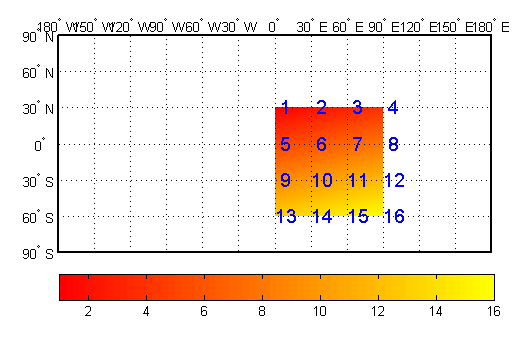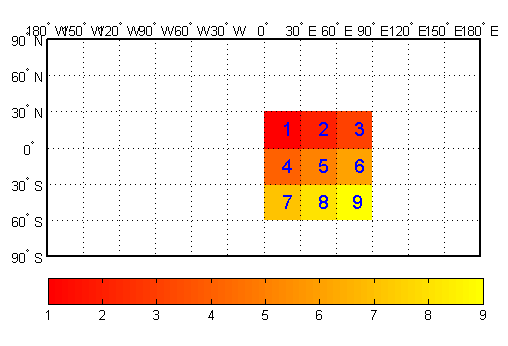## Geographic Interpretations of Geolocated Grids

Mapping Toolbox™ software supports three different interpretations of geolocated data grids:

• First, a map matrix having the same number of rows and columns as the latitude and longitude coordinate matrices represents the values of the map data at the corresponding geographic points (centers of data cells).

• Next, a map matrix having one fewer row and one fewer column than the geographic coordinate matrices represents the values of the map data within the area formed by the four adjacent latitudes and longitudes.

• Finally, if the latitude and longitude matrices have smaller dimensions than the map matrix, you can interpret them as describing a coarser graticule, or mesh of latitude and longitude cells, into which the blocks of map data are warped.

This section discusses the first two interpretations of geolocated data grids. For more information on the use of graticules, see The Map Grid.

### Type 1: Values Associated with the Upper Left Grid Coordinate

As an example of the first interpretation, consider a 4-by-4 map matrix whose cell size is 30-by-30 degrees, along with its corresponding 4-by-4 latitude and longitude matrices:

```Z = [ ... 1 2 3 4; ... 5 6 7 8; ... 9 10 11 12; ... 13 14 15 16]; lat = [ ... 30 30 30 30; ... 0 0 0 0; ... -30 -30 -30 -30; ... -60 -60 -60 -60]; lon = [ ... 0 30 60 90;... 0 30 60 90;... 0 30 60 90;... 0 30 60 90]; ```

Display the geolocated data grid with the values of `map` shown at the associated latitudes and longitudes:

```figure('Color','white','Colormap',autumn(64)) axesm('pcarree','Grid','on','Frame','on',... 'PLineLocation',30,'PLabelLocation',30) mlabel; plabel; axis off; tightmap h = geoshow(lat,lon,Z,'DisplayType','surface'); set(h,'ZData',zeros(size(Z))) ht = textm(lat(:),lon(:),num2str(Z(:)), ... 'Color','blue','FontSize',14); colorbar('southoutside') ```Notice that only 9 of the 16 total cells are displayed. The value displayed for each cell is the value at the upper left corner of that cell, whose coordinates are given by the corresponding `lat` and `lon` elements. By convention, the last row and column of the map matrix are not displayed, although they exist in the `CData` property of the surface object.

### Type 2: Values Centered Within Four Adjacent Coordinates

For the second interpretation, consider a 3-by-3 map matrix with the same `lat` and `lon` variables:

```delete(h) delete(ht) Z3by3 = [ ... 1 2 3; ... 4 5 6; ... 7 8 9]; h = geoshow(lat,lon,Z3by3,'DisplayType','texturemap'); tlat = [ ... 15 15 15; ... -15 -15 -15; ... -45 -45 -45]; tlon = [ ... 15 45 75; ... 15 45 75; ... 15 45 74]; textm(tlat(:),tlon(:),num2str(Z3by3(:)), ... 'Color','blue','FontSize',14) ```

Display a surface plot of the map matrix, with the values of `map` shown at the center of the associated cells:All the map data is displayed for this geolocated data grid. The value of each cell is the value at the center of the cell, and the latitudes and longitudes in the coordinate matrices are the boundaries for the cells.

### Ordering of Cells

You may have noticed that the first row of the matrix is displayed as the top of the map, whereas for a regular data grid, the opposite was true: the first row corresponded to the bottom of the map. This difference is entirely due to how the `lat` and `lon` matrices are ordered. In a geolocated data grid, the order of values in the two coordinate matrices determines the arrangement of the displayed values.

### Transform Regular to Geolocated Grids

When required, a regular data grid can be transformed into a geolocated data grid. This simply requires that a pair of coordinate matrices be computed at the desired spatial resolution from the regular grid. For example, load elevation raster data and a geographic cells reference object. Then, create a latitude-longitude grid for the data by calling the `geographicGrid` function.

```load topo60c [lat,lon] = geographicGrid(topo60cR); ```

### Transforming Geolocated to Regular Grids

Conversely, a regular data grid can also be constructed from a geolocated data grid. The coordinates and values can be embedded in a new regular data grid. The function that performs this conversion is `geoloc2grid`; it takes a geolocated data grid and a cell size as inputs.

## SupportGet trial now